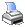Navigation:  Reference > Commands > ForThis is the first part of the FOR/NEXT loop.  In this part of the command you can specify the counter, start value, stop value, and the amount to add to or subtract from the counter each time the loop is executed.

FOR(

 counter - fn Required - The name of the field used as a loop counter.  This can be any integer field (B, I or R).

start_value - f/c/e Required - The first value to be used in the loop.  The counter field is initialized to this value the first time through.

stop_value - f/c/e Required - When the counter reaches this value the program knows to exit the loop at the NEXT command.

step_value - f/c/e   Required - How much to add to or subtract from counter each time through the loop.  If you wish to subtract (or decrement) counter in the loop, precede the value with the minus sign.  For example, a step_value of -1 will reduce the counter value by 1 each time the loop is executed.

)

All fields/values in the FOR command except counter must be integers (B, I or R).

NOTE:  If the start_value is greater than the stop_value (or less than, if the step_value is a negative number) then the loop will not execute even once.

NOTE:  Don't forget the parentheses around the values and the values themselves are separated by semi-colons; for example:

FOR(x;1;10;1) // this would count up from 1 to 10

//put code here

msg X

NEXT

OR

FOR(X;10;1;-1) // this would count Down from 10 -1

//put code here

msg X

NEXT

For more information on the different structured programming commands, and the FOR command in particular, please see Structured Programming Commands.